# Power trend equation and forecast

Excel 365
If you have data with only positive values that show a steady increase or decrease with a fixed rate of growth or decline, use the power trendline. See more about the different types of trendlines you can create in Excel: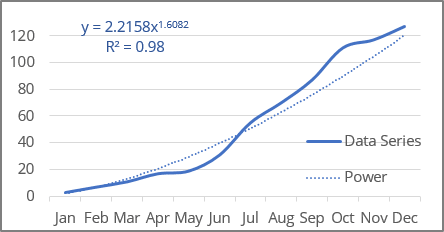Excel uses the least squares method to fit the curve to the data points.

For making a trend line, two sets of variables are required:

• an independent, predictor, or explanatory variable x (named as known_x's),
• a dependent or response variable y (named as known_y's).

## Power trendline equation and formulas

The power trendline is a curved line described by the function:

y = a * xb

where:

• a and b are the parameters of the function found by the least squares method (also named function coefficients or constants).

Note: Excel transforms the power function approximation into the linear model fitting: the power function y = a * xb is transformed to a linear function by a change of variables. After changing the variables Y = ln (y), X = ln (x) and A = ln (a), the calculations become similar to the linear case Y = b * X + A. To find parameter a, it is necessary to perform the inverse transformation a = EXP (A):

Excel offers the following functions to calculate the trend parameters:

Using LINEST function:

• a: = EXP (INDEX (LINEST (LN (known_y's), LN (known_x's)), 1, 2))
• b: = INDEX (LINEST (LN (known_y's), LN (known_x's)), 1)

Using LOGEST function:

• a: = INDEX (LOGEST (known_y's, LN (known_x's)), 1, 2))
• b: = LN (INDEX (LOGEST (known_y's, LN (known_x's)), 1)

Note: You can mix calls to these functions to get different parameters, but the results are still the same. For example:

• a: = INDEX (LOGEST (known_y's, LN (known_x's)), 1, 2))
• b: = INDEX (LINEST (LN (known_y's), LN (known_x's)), 1)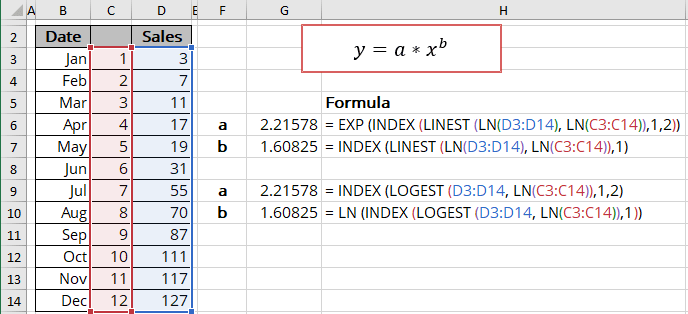The EXP () function returns the result of the constant e raised to the power of a number:

= EXP (number)

where:

• number (required) is a power that e is raised to.

The LN () function is the inverse of the EXP () function and returns the natural logarithm of a given number:

= LN (number)

where:

• number (required) is a number to take the natural logarithm of.

The array function LINEST () calculates the linear trend that fits best the known data and then returns an array with statistics describing the trend line:

= LINEST (known_y's, [known_x's], [const], [stats])

The array function LOGEST () calculates the power curve that fits best the known data and then returns an array with statistics describing the trend line:

= LOGEST (known_y's, [known_x's], [const], [stats])

where:

• known_y's (required) are dependent data values,
• known_x's (optional) are independent data values:

These variables cannot be omitted for the correct calculation of a power trend. In any case, you should provide the known_x's or array {1,2,3,...} that has the same size as known_y's,

• const (optional) is a Boolean value specifying whether to force the parameter a to equal 1:
• If const is TRUE or omitted, the parameter a is calculated normally,
• If const is FALSE, the parameter a is equal to 1, so the function will look like y = xb.
• stats (optional) is a Boolean value specifying whether to return additional regression statistics:
• If stats is TRUE, the function returns the additional regression statistics in the form of an array: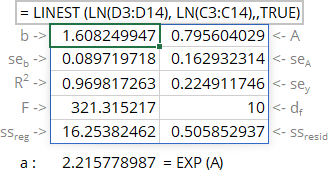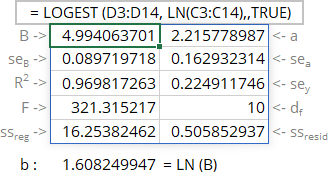where:

Line Statistics Description
1 b a Parameters of the function
2 seb sea Standard error of parameters
3 R2 R-squared value
sey Standard error of y estimate (also known as Standard Error of a regression) shows how big the prediction error is
4 F F statistic (F-observed value)
dr Degrees of freedom
5 ssreg Regression sum of squares (also known as SSR)
ssresid Residual sum of squares (also known as SSE)
• If stats is FALSE or omitted, the function returns only the calculated parameters.

Notes:

1. If you have a version of Microsoft Excel other than Microsoft 365, functions that return arrays must be entered as array functions using Ctrl+Shift+Enter unless you can just press Enter.
2. Because the LINEST () and LOGEST () functions return an array of data, you can use the INDEX () function to display only the desired parameter(s) from the returned data array.
3. Because the statistics are calculated for the linear model, you see different values in statistics and on the chart. So, some statistics seem incorrect for the power functions.

## Calculating the power trendline values

To calculate the power trendline values, Excel offers the array function GROWTH () (see the notes above about array functions) that returns values for the corresponding power function:

= GROWTH (known_y's, [known_x's], [new_x's], [const])

where:

• known_y's (required) are dependent data values,
• known_x's (optional) are independent data values:

These variables cannot be omitted for the correct calculation for a power trend. In any case, you should provide the known_x's or array {1,2,3,...} that has the same size as known_y's,

• new_x's (optional) are the new x-values for which the GROWTH () function should calculate corresponding y-values:
• If you omit new_x's, it is assumed to be the same as known_x's,
• const (optional) is a Boolean value specifying whether to force the parameter a to equal 1:
• If const is TRUE or omitted, the parameter a is calculated normally,
• If const is FALSE, the parameter a is set to 1.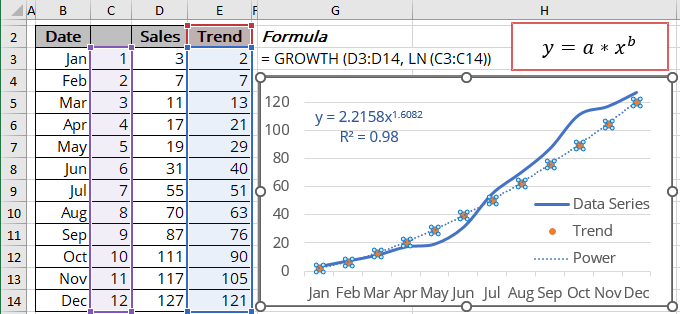Note: You can get the same results using the function TREND () as for linear trend with the following transformation:

= EXP (TREND (LN (known_y's), LN (known_x's)))

## R-squared value

Using the linear model to determine function parameters works well, but some statistics that return the LINEST () and LOGEST () functions, such as the R-squared value, diverge! You can see different values in the statistics array and on the chart.

The R-squared value (in Excel, R2) determines how well the trendline fits the data. The R-squared value can vary from 0 to 1: the nearer R2 is to 1, the better the trendline fits the data.

The R-squared value displayed on the chart (see how to turn on the R-squared value) is the Pearson product-moment correlation coefficient (approximation R2 is the coefficient of determination):

• The RSQ () function returns the R-squared value of two data sets:

= RSQ (array1, array2)

where array1 and array2 (both are required) are two data sets: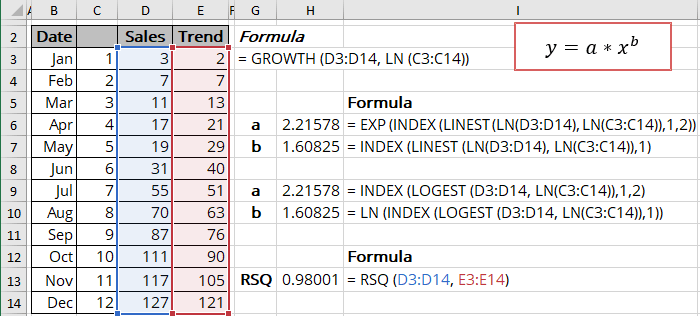Notes: The value you see in the LINEST () and LOGEST () statistics is the R-squared value between the logarithms of array 1 and array 2. In this example: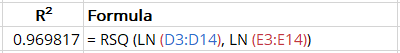## Forecast using the power trendline

Using the GROWTH () function, you can calculate the predicted values y for the new_x's (don't forget to add the natural logarithm function for the new_x's):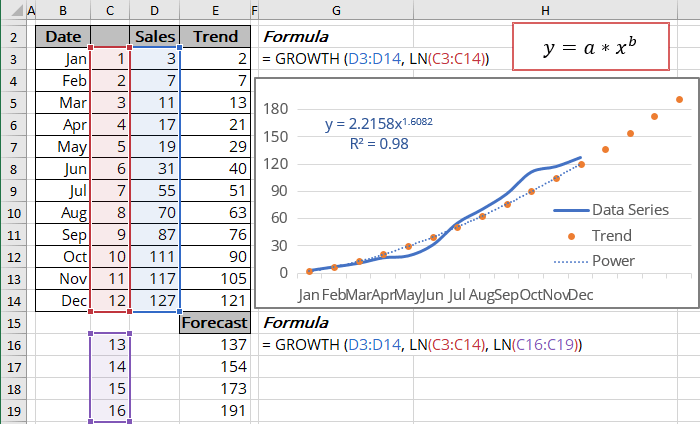Note: You can get the same results using the function TREND () as for linear trend with the following transformation:

= EXP (TREND (LN (known_y's), LN (known_x's), LN (new_x's)))